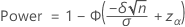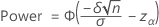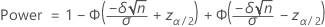# Methods and formulas for Power and Sample Size for 1-Sample Z

Select the method or formula of your choice.

## Calculating power

### One-sided power (H1: μ > μ0)### One-sided power (H1: μ < μ0)### Two-sided power (H1: μ ≠ μ0)### Notation

TermDescription
μ true value of the population mean
μ 0 hypothesized value of the population mean
Φcumulative distribution function of the standard normal distribution
δ difference between the true mean and the hypothesized mean
n sample size
σ population standard deviation
z α one-sided critical value (upper α point of the standard normal distribution)
z α/2 two-sided critical value (upper α/2 point of the standard normal distribution)
α significance level

## Calculating sample size and difference

If you provide values for power and sample size, Minitab calculates the value of the difference. If you provide values for power and difference, Minitab calculates the value of the sample size.

### Two-sided method

Minitab uses an iterative algorithm with the power equation. At each iteration, Minitab evaluates the power for a trial sample size or trial difference value, and stops when the power function equals a target power value.

### One-sided method

Minitab solves directly for the value you request with the following equations:

### Target power and actual power

When Minitab calculates sample size, it may find that no integer value of sample size yields your target power. In such cases, Minitab displays the target value for power alongside the actual power. Actual power is a value corresponding to an integer sample size, and which is nearest to, yet greater than, the target value.

### Notation

TermDescription
n sample size
σ standard deviation
zα one-sided critical value (upper α point of the standard normal distribution)
α significance level
zβ upper β point of the standard normal distribution
β 1 – power
δ difference between the population mean and the hypothesized mean